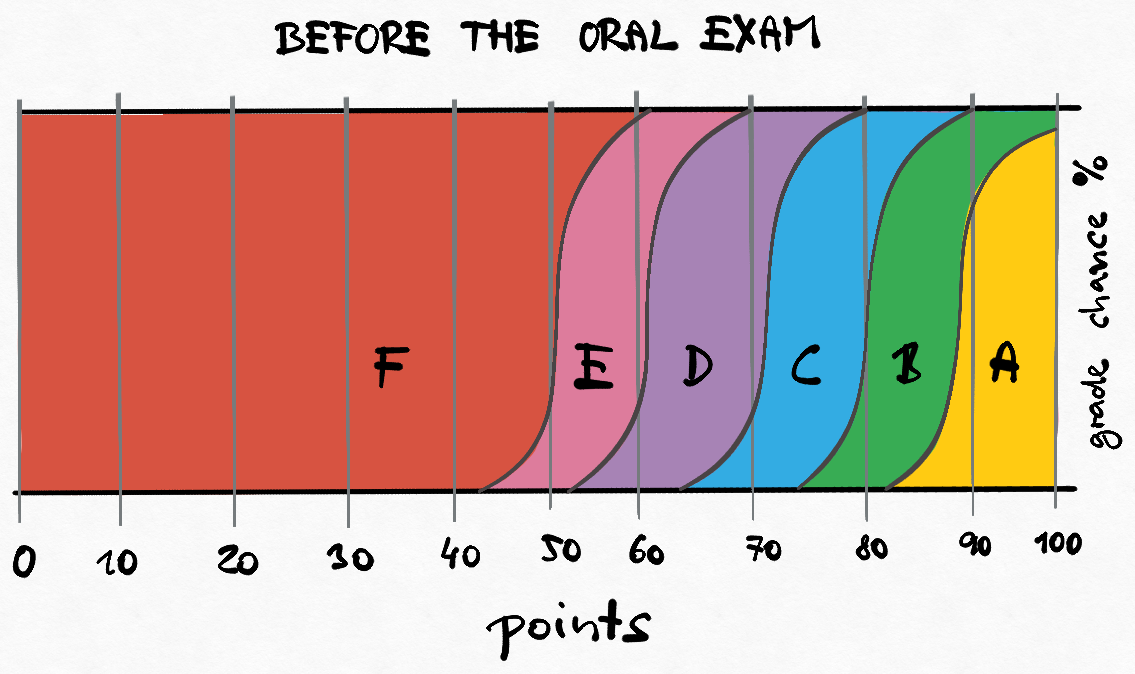Warning

## Summary

This course introduces statistical decision theory and surveys canonical and advanced classifiers such as perceptrons, AdaBoost, support vector machines, and neural nets.

## Basic info

Winter semester 2021/2022

Where and when: KN:E-126 at Building G, Karlovo namesti, Monday 12:45-14:15

Teaching: Jiří Matas (JM) matas@cmp.felk.cvut.cz, Ondřej Drbohlav (OD) drbohlav@cmp.felk.cvut.cz

## Lecture plan 2021/2022

 Week Date Lect. Slides Topic Wiki Additional material 1 20.9. JM pdf Introduction. Basic notions. The Bayesian recognition problem Machine_learning Naive_Bayes_classifier some simple problems 2 27.9. JM pdf Non-Bayesian tasks Minimax 3 4.10. JM pdf Parameter estimation of probabilistic models. Maximum likelihood method Maximum_likelihood 4 11.10. JM pdf Nearest neighbour method. Non-parametric density estimation. K-nearest_neighbor_algorithm 5 18.10. JM pdf Logistic regression Logistic_regression 6 25.10. JM pdf Classifier training. Linear classifier. Perceptron. Linear_classifier Perceptron 7 1.11. JM pdf SVM classifier Support_vector_machine 8 8.11. JM pdf Adaboost learning Adaboost 9 15.11. JM pdf Neural networks. Backpropagation Artificial_neural_network 10 22.11. JM pdf Cluster analysis, k-means method K-means_clustering K-means++ 11 29.11. JM pdf EM (Expectation Maximization) algorithm. Expectation_maximization_algorithm Hoffmann,Bishop, Flach 12 6.12. JM pdf Feature selection and extraction. PCA, LDA. Principal_component_analysis Linear_discriminant_analysis Optimalizace (CZ): PCA slides, script 7.2 13 13.12. JM pdf Decision trees. Decision_tree Decision_tree_learning Rudin@MIT 14 3.1. JM Basic notions recapitulation, links between methods, answers to exam questions )
• Duda R.O., Hart, P.E.,Stork, D.G.: Pattern Classification, John Willey and Sons, 2nd edition, New York, 2001
• Schlesinger M.I., Hlaváč V.: Ten Lectures on Statistical and Structural Pattern Recognition, Springer, 2002
• Bishop, C.: Pattern Recognition and Machine Learning, Springer, 2011
• Goodfellow, I., Bengio, Y. and Courville, A.: Deep Learning, MIT Press, 2016. www

## Assessment (zápočet)

Conditions for assessment are in the lab section.

## Exam

• Only students who receive all credits from the lab work and are granted the assessment (“zápočet”) can be examined.
• The labs contribute 50% to your final evaluation, the written part of the exam contributes 40% and the oral part contributes 10%.
• A threshold for passing the exam is set, usually between 5-10 points (out of 40), depending on the complexity of the test.
• Your grade chances after the written exam test and before the oral exam are illustrated in the image below. Beware: The scheme is approximate only, but illustrates how the oral exam influences the final grade.
• The questions used in the test are available here (if one can solve these questions, one will likely do well on the exam)
• Oral part starts approximately 2 hours after the end of the test (the interim time is used to correct the tests), or if the number of students is large, the following day.. It contributes to the final evaluation by 10%.
• The oral part is compulsory!
• Example oral exam questions are available here.